# Plancherel theorem

(Redirected from Parseval-Plancherel formula)

For any square-summable function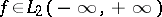the integral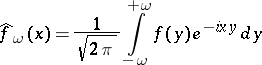converges in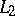to some function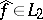as, i.e.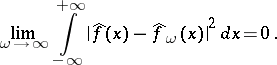(1)

Here the functionitself is representable as the limit inof the integralsas, i.e.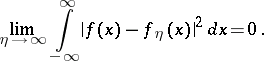Also, the following relation holds: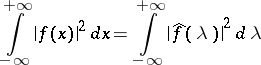(the Parseval–Plancherel formula).

The function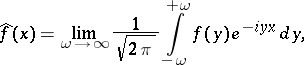where the limit is understood in the sense of convergence in(as in (1)), is called the Fourier transform of; it is sometimes denoted by the symbolic formula:(2)

where the integral in (2) must be understood in the sense of the principal value atin the metric of. One similarly interprets the equation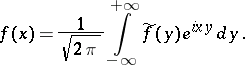(3)

For functions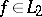, the integrals (2) and (3) exist in the sense of the principal value for almost all.

The functionsand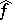also satisfy the following equations for almost-all: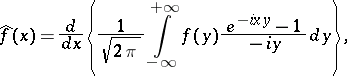If Fourier transformation is denoted by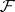and if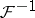denotes the inverse, then Plancherel's theorem can be rephrased as follows:andare mutually-inverse unitary operators on(cf. Unitary operator).

The theorem was established by M. Plancherel (1910).